特征列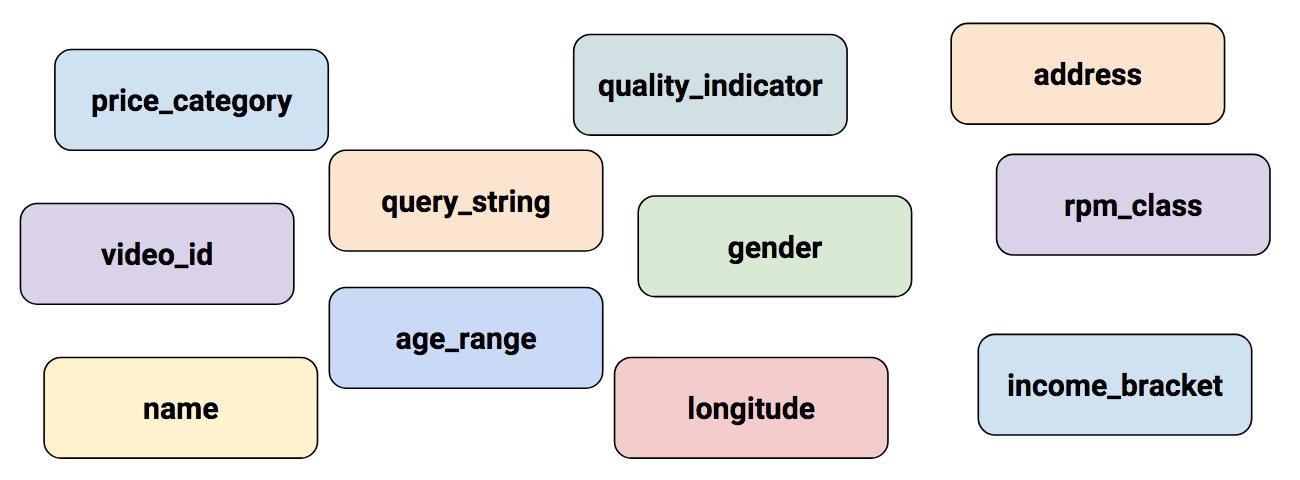深度神经网络的输入

• kitchenware
• electronics
• sports

• 0kitchenware 不存在
• 0electronics 不存在
• 1sports 存在

特征列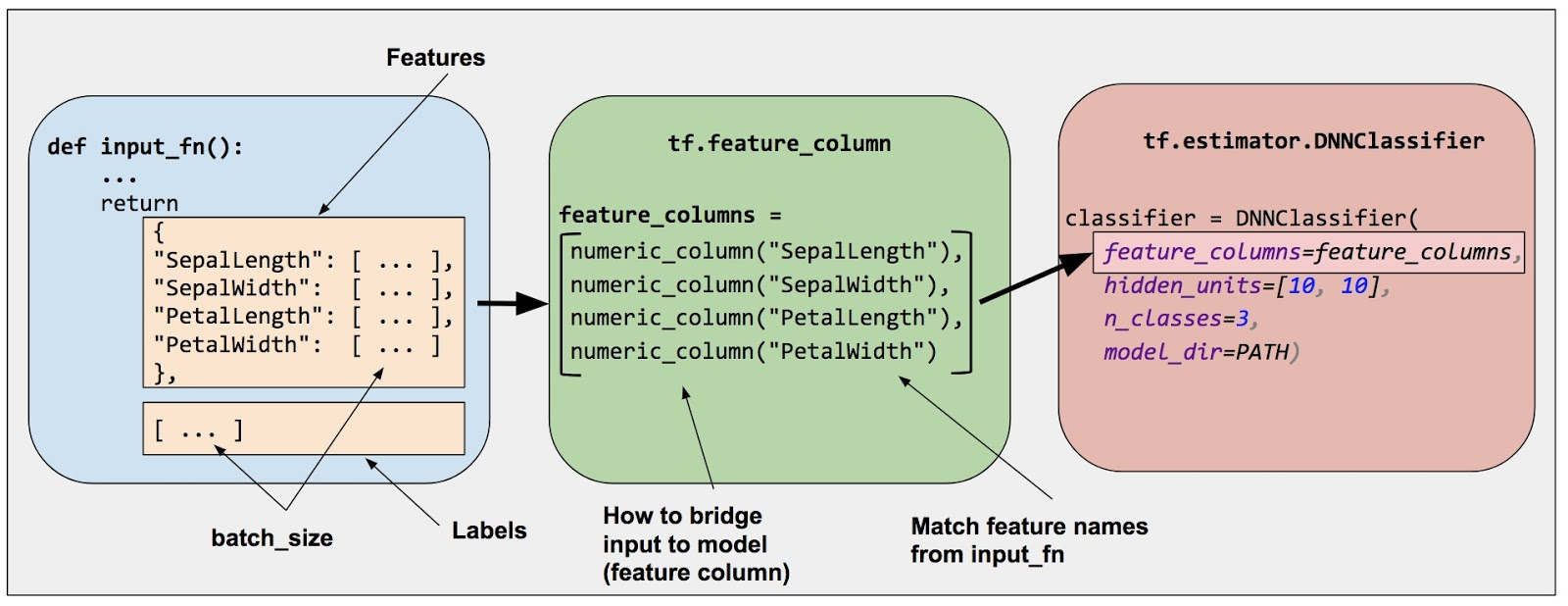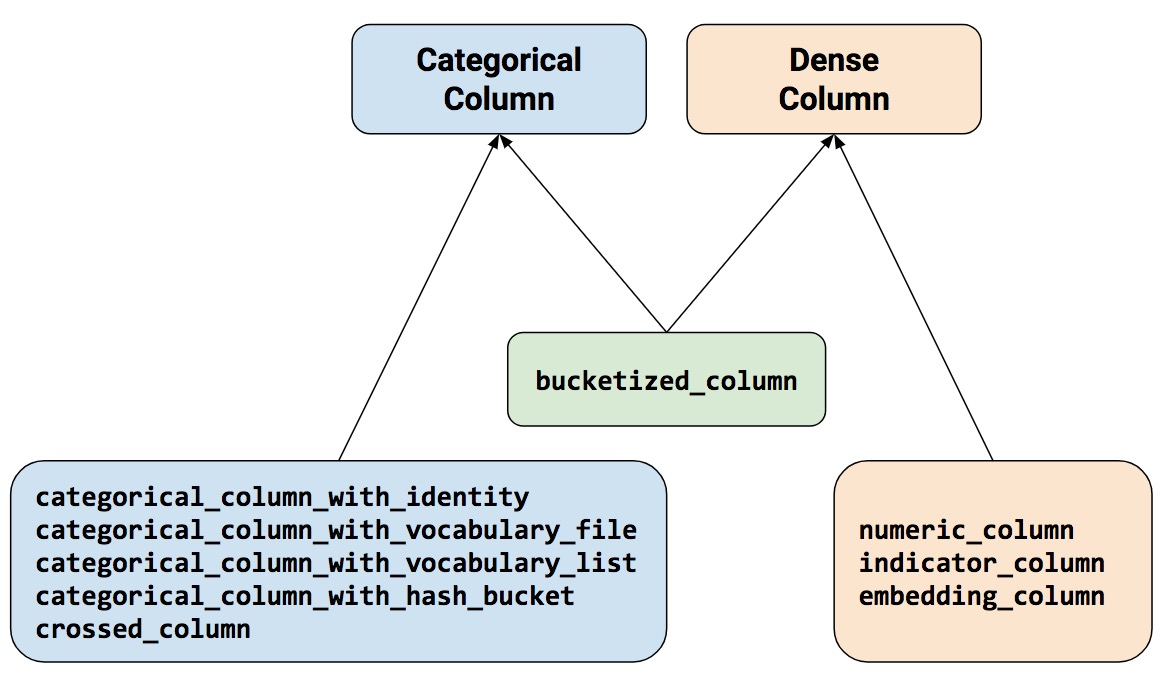数值列

• SepalLength
• SepalWidth
• PetalLength
• PetalWidth

# Defaults to a tf.float32 scalar.
numeric_feature_column = tf.feature_column.numeric_column(key="SepalLength")


# Represent a tf.float64 scalar.
numeric_feature_column = tf.feature_column.numeric_column(key="SepalLength",
dtype=tf.float64)


# Represent a 10-element vector in which each cell contains a tf.float32.
vector_feature_column = tf.feature_column.numeric_column(key="Bowling",
shape=10)

# Represent a 10x5 matrix in which each cell contains a tf.float32.
matrix_feature_column = tf.feature_column.numeric_column(key="MyMatrix",
shape=[10,5])


分桶列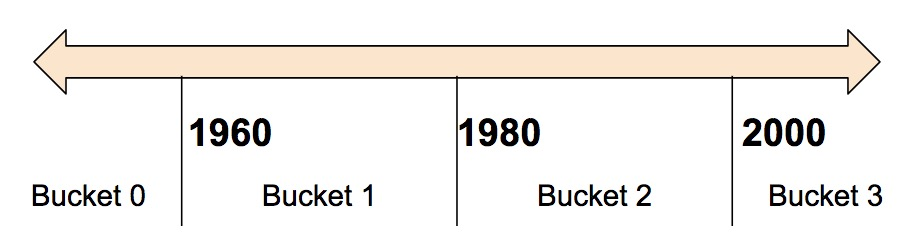< 1960 年 [1, 0, 0, 0]
>= 1960 年但 < 1980 年 [0, 1, 0, 0]
>= 1980 年但 < 2000 年 [0, 0, 1, 0]
>= 2000 年 [0, 0, 0, 1]

# First, convert the raw input to a numeric column.
numeric_feature_column = tf.feature_column.numeric_column("Year")

# Then, bucketize the numeric column on the years 1960, 1980, and 2000.
bucketized_feature_column = tf.feature_column.bucketized_column(
source_column = numeric_feature_column,
boundaries = [1960, 1980, 2000])


分类标识列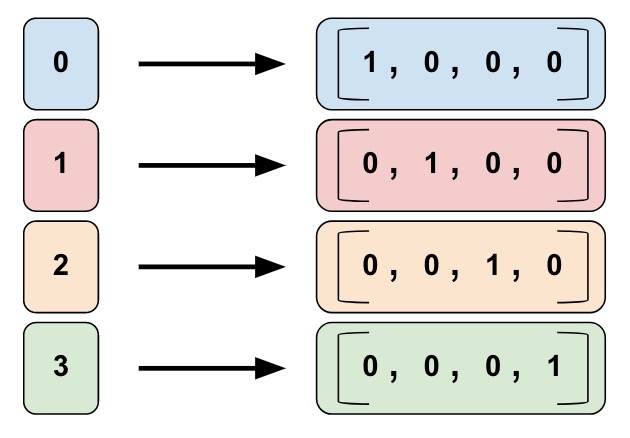• 0="kitchenware"
• 1="electronics"
• 2="sport"
# Create categorical output for an integer feature named "my_feature_b",
# The values of my_feature_b must be >= 0 and < num_buckets
identity_feature_column = tf.feature_column.categorical_column_with_identity(
key='my_feature_b',
num_buckets=4) # Values [0, 4)

# In order for the preceding call to work, the input_fn() must return
# a dictionary containing 'my_feature_b' as a key. Furthermore, the values
# assigned to 'my_feature_b' must belong to the set [0, 4).
def input_fn():
...
return ({ 'my_feature_a':[7, 9, 5, 2], 'my_feature_b':[3, 1, 2, 2] },
[Label_values])


分类词汇列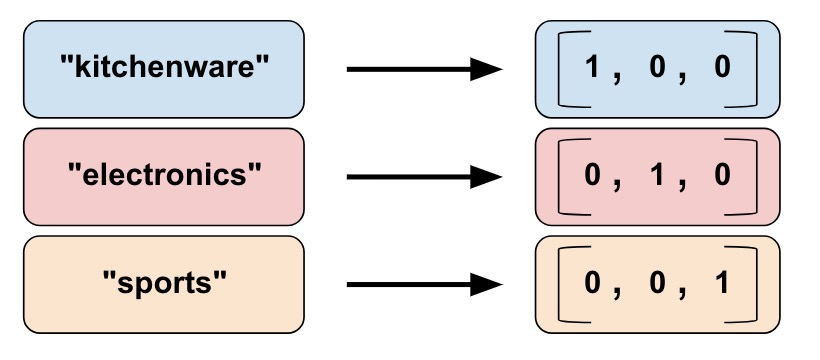categorical_column_with_vocabulary_list 根据明确的词汇表将每个字符串映射到一个整数。例如：

# Given input "feature_name_from_input_fn" which is a string,
# create a categorical feature by mapping the input to one of
# the elements in the vocabulary list.
vocabulary_feature_column =
tf.feature_column.categorical_column_with_vocabulary_list(
key=feature_name_from_input_fn,
vocabulary_list=["kitchenware", "electronics", "sports"])



# Given input "feature_name_from_input_fn" which is a string,
# create a categorical feature to our model by mapping the input to one of
# the elements in the vocabulary file
vocabulary_feature_column =
tf.feature_column.categorical_column_with_vocabulary_file(
key=feature_name_from_input_fn,
vocabulary_file="product_class.txt",
vocabulary_size=3)


product_class.txt 中的每个词汇元素应各占一行。在我们的示例中：

kitchenware
electronics
sports


经过哈希处理的列

# pseudocode
feature_id = hash(raw_feature) % hash_buckets_size


hashed_feature_column =
tf.feature_column.categorical_column_with_hash_bucket(
key = "some_feature",
hash_buckets_size = 100) # The number of categories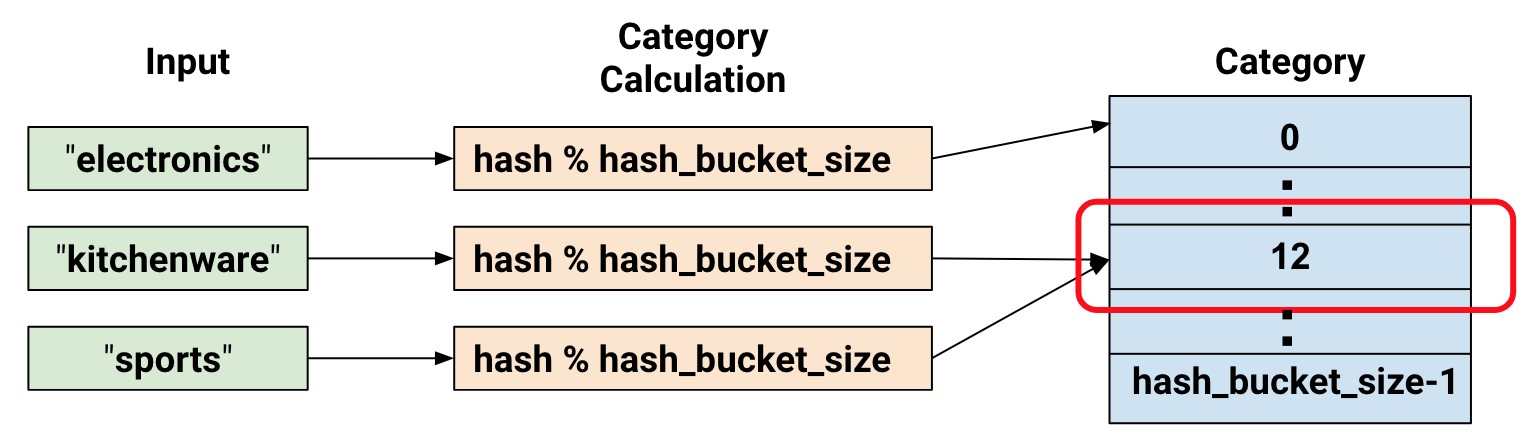组合列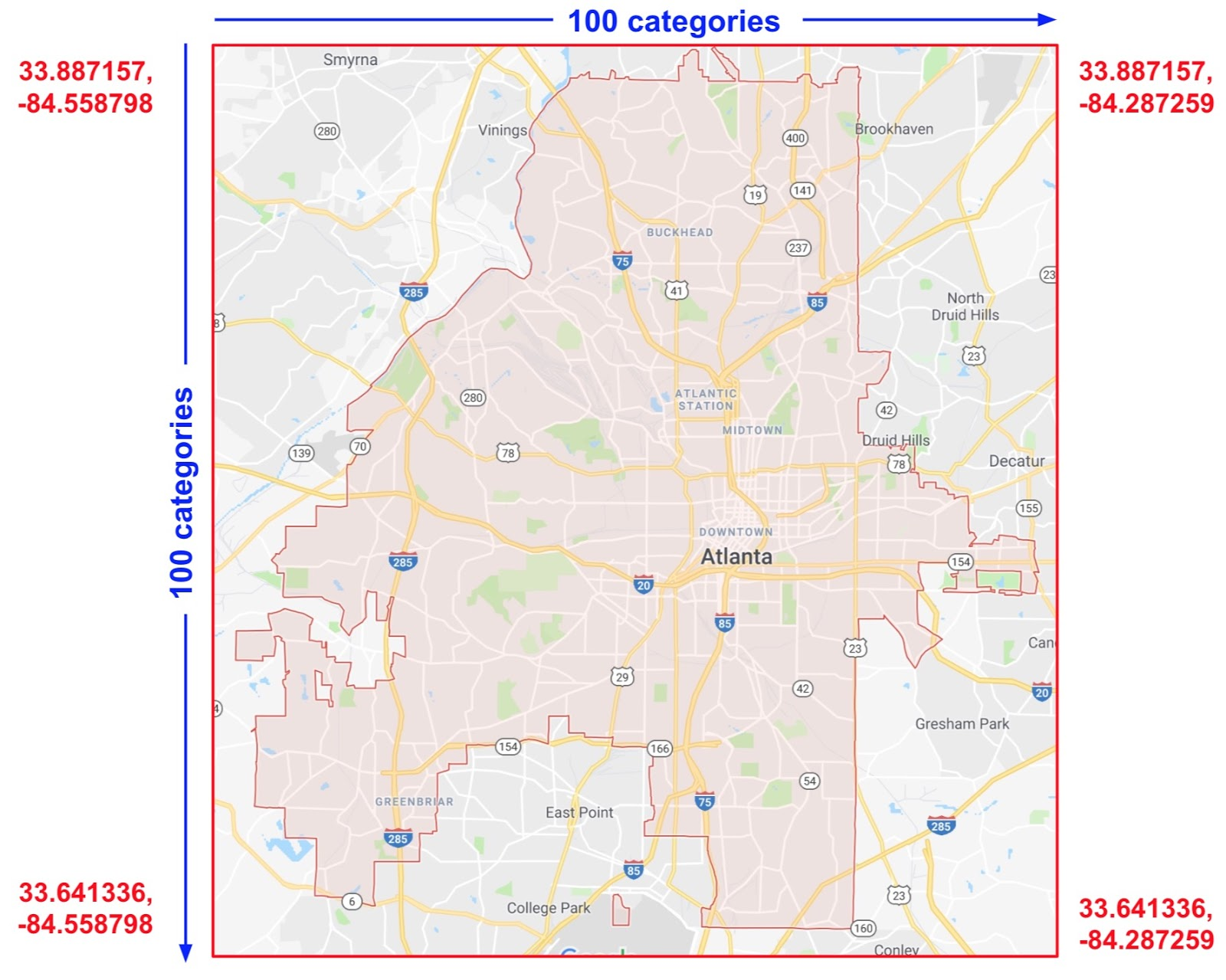def make_dataset(latitude, longitude, labels):
assert latitude.shape == longitude.shape == labels.shape

features = {'latitude': latitude.flatten(),
'longitude': longitude.flatten()}
labels=labels.flatten()

return tf.data.Dataset.from_tensor_slices((features, labels))

# Bucketize the latitude and longitude using the edges
latitude_bucket_fc = tf.feature_column.bucketized_column(
tf.feature_column.numeric_column('latitude'),
list(atlanta.latitude.edges))

longitude_bucket_fc = tf.feature_column.bucketized_column(
tf.feature_column.numeric_column('longitude'),
list(atlanta.longitude.edges))

# Cross the bucketized columns, using 5000 hash bins.
crossed_lat_lon_fc = tf.feature_column.crossed_column(
[latitude_bucket_fc, longitude_bucket_fc], 5000)

fc = [
latitude_bucket_fc,
longitude_bucket_fc,
crossed_lat_lon_fc]

# Build and train the Estimator.
est = tf.estimator.LinearRegressor(fc, ...)


• 特征名称；也就是 input_fn 返回的 dict 中的名称。
• 任意分类列，categorical_column_with_hash_bucket 除外（因为 crossed_column 会对输入进行哈希处理）。

 (0,0),  (0,1)...  (0,99)
(1,0),  (1,1)...  (1,99)
...     ...       ...
(99,0), (99,1)...(99, 99)


指标列和嵌入列categorical_column = ... # Create any type of categorical column.

# Represent the categorical column as an indicator column.
indicator_column = tf.feature_column.indicator_column(categorical_column)


• "dog"
• "spoon"
• "scissors"
• "guitar"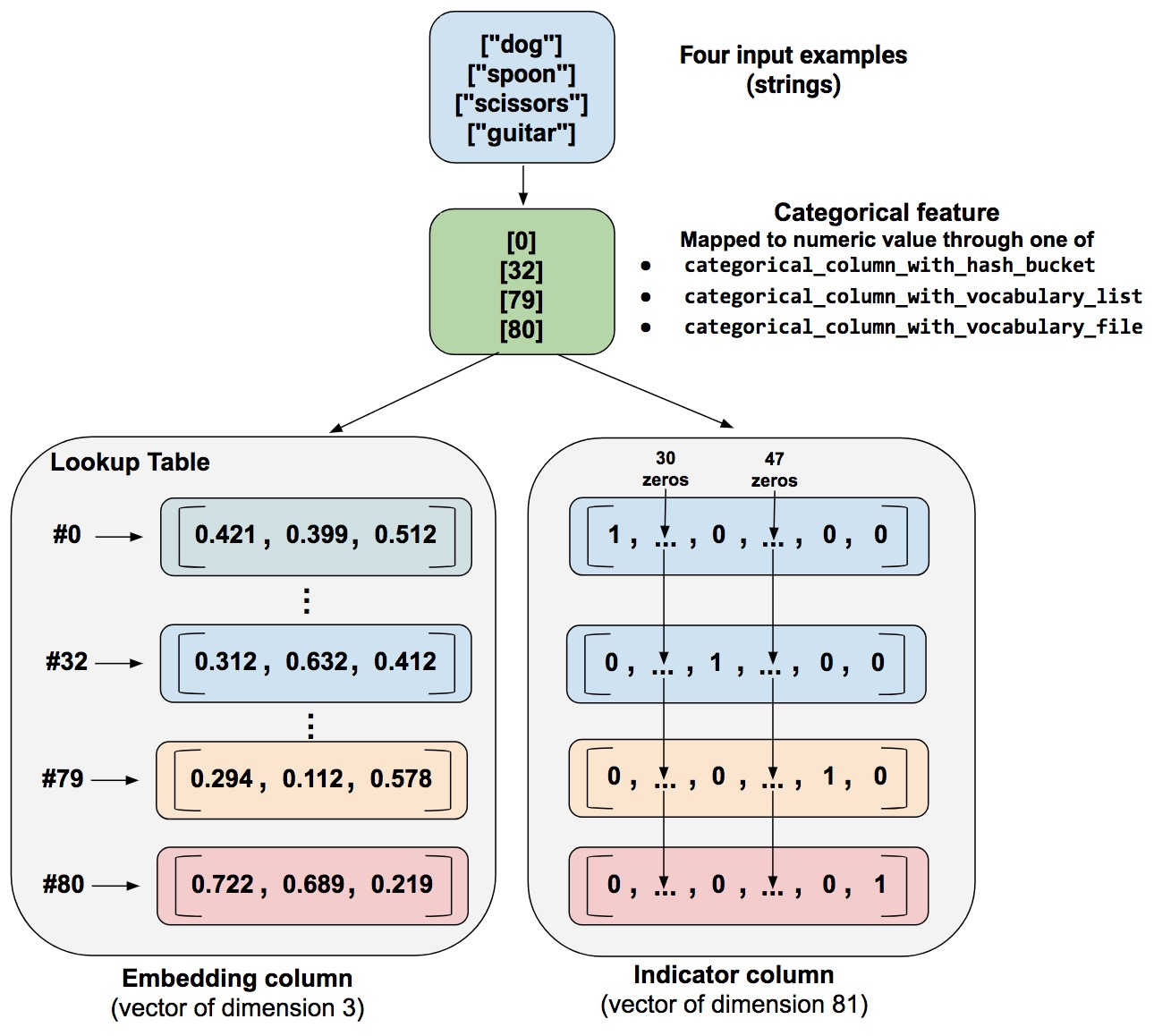• 作为指标列。函数将每个分类数值转换为一个 81 元素的矢量（因为我们的词汇表由 81 个字词组成），将 1 置于分类值 (0, 32, 79, 80) 的索引处，将 0 置于所有其他位置。

• 作为嵌入列。函数将分类数值 (0, 32, 79, 80) 用作对照表的索引。该对照表中的每个槽位都包含一个 3 元素矢量。

embedding_dimensions =  number_of_categories**0.25


3 =  81**0.25


categorical_column = ... # Create any categorical column

# Represent the categorical column as an embedding column.
# This means creating an embedding vector lookup table with one element for each category.
embedding_column = tf.feature_column.embedding_column(
categorical_column=categorical_column,
dimension=embedding_dimensions)